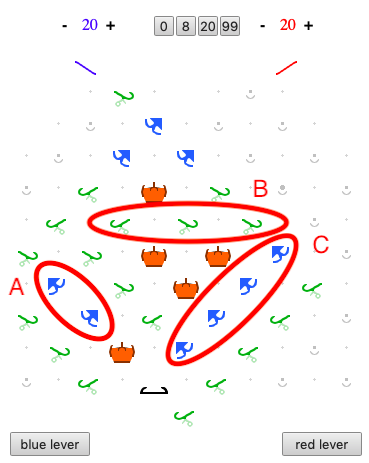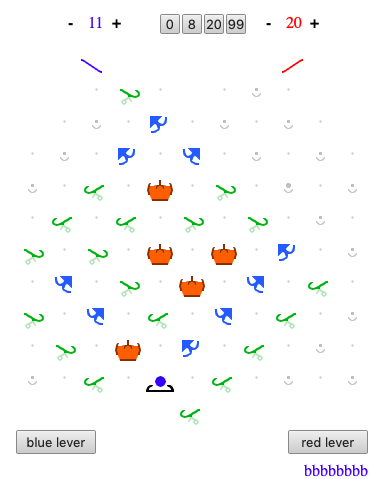# Variations on Puzzle 56

After solving Puzzle 56 “Complete Multiplication” I was inspired to create my own multipliers, which are improvements in some way over the solution in the book. Note that I am not claiming to have found alternate solutions to Puzzle 56 itself.
I have successfully completed the following two challenges. I will post them here and present my solutions later. The only restriction is that solutions must use only what is supplied with Turing Tumble as it now exists.

Challenge 1: The problem posed in 56 is to multiply a binary number stored in a first 2-bit register by another number stored in a second 2-bit register, with the result appearing in a in a third, 3-bit register. (By “bit” I mean binary-digit in the general sense, not the arrow-shaped component in TT.) Create a multiplier that solves the same problem as in Puzzle 1 but in an improved way. “Improved” can mean, as examples, using fewer parts or multiplying faster, i.e. using fewer steps. (Note that the book solution to 56 has a wasted step.)

Challenge 2: Create a multiplier that multiplies a number in a 2-bit register by a number in a 3-bit register, with the result appearing in a 4-bit register.

Challenge 2 (based on DigiComp II):

A x B = CFeatured: A = 2, B = 3, C= 2 x 3 = 6